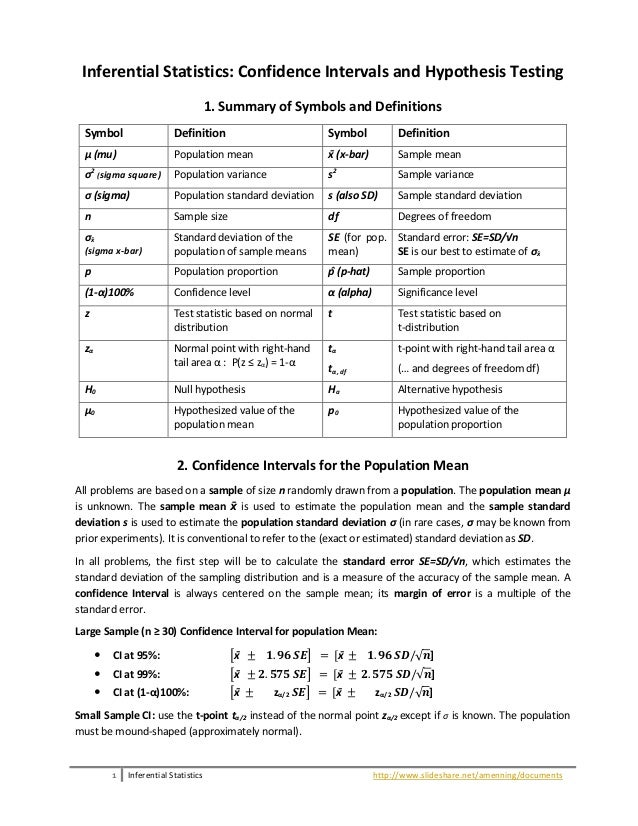Successfully reported this slideshow.
We use your LinkedIn profile and activity data to personalize ads and to show you more relevant ads. You can change your ad preferences anytime.Upcoming SlideShare
×

# Summary of Inferential Statistics - Handout

1,616 views

Published on

Summary of Symbols and Definitions; Confidence Intervals for the Population Mean; Confidence Interval and Hypothesis Testing for Sample Mean and Proportion

Published in: Economy & Finance
• Full Name
Comment goes here.

Are you sure you want to Yes No
Your message goes here• Be the first to comment

### Summary of Inferential Statistics - Handout

1. 1. 1 Inferential Statistics http://www.slideshare.net/amenning/documents Inferential Statistics: Confidence Intervals and Hypothesis Testing 1. Summary of Symbols and Definitions Symbol Definition Symbol Definition μ (mu) Population mean x̄ (x-bar) Sample mean σ2 (sigma square) Population variance s2 Sample variance σ (sigma) Population standard deviation s (also SD) Sample standard deviation n Sample size df Degrees of freedom σx̄ (sigma x-bar) Standard deviation of the population of sample means SE (for pop. mean) Standard error: SE=SD/√n SE is our best to estimate of σx̄ p Population proportion p̂ (p-hat) Sample proportion (1-α)100% Confidence level α (alpha) Significance level z Test statistic based on normal distribution t Test statistic based on t-distribution zα Normal point with right-hand tail area α : P(z ≤ zα) = 1-α tα tα, df t-point with right-hand tail area α (… and degrees of freedom df) H0 Null hypothesis Ha Alternative hypothesis μ0 Hypothesized value of the population mean p0 Hypothesized value of the population proportion 2. Confidence Intervals for the Population Mean All problems are based on a sample of size n randomly drawn from a population. The population mean μ is unknown. The sample mean x̄ is used to estimate the population mean and the sample standard deviation s is used to estimate the population standard deviation σ (in rare cases, σ may be known from prior experiments). It is conventional to refer to the (exact or estimated) standard deviation as SD. In all problems, the first step will be to calculate the standard error SE=SD/√n, which estimates the standard deviation of the sampling distribution and is a measure of the accuracy of the sample mean. A confidence Interval is always centered on the sample mean; its margin of error is a multiple of the standard error. Large Sample (n ≥ 30) Confidence Interval for population Mean:  CI at 95%: [x̄ ± 𝟏. 𝟗𝟔 𝑺𝑬] = [x̄ ± 𝟏. 𝟗𝟔 𝑺𝑫/√ 𝒏]  CI at 99%: [x̄ ± 𝟐. 𝟓𝟕𝟓 𝑺𝑬] = [x̄ ± 𝟐. 𝟓𝟕𝟓 𝑺𝑫/√ 𝒏]  CI at (1-α)100%: [x̄ ± zα/2 𝑺𝑬] = [x̄ ± zα/2 𝑺𝑫/√ 𝒏] Small Sample CI: use the t-point tα/2 instead of the normal point zα/2 except if σ is known. The population must be mound-shaped (approximately normal).
2. 2. 2 Inferential Statistics http://www.slideshare.net/amenning/documents 3. Confidence Interval and Hypothesis Testing for Sample Mean and Proportion Sample Mean Sample Proportion Basics Estimate x̄ p̂ Conditions (valid if) n ≥ 30 or population distribution normal (mound-shaped) np̂ ≥ 5 and n(1-p̂) ≥ 5 Standard error 𝑺𝑬 = 𝑺𝑫/√ 𝒏 Substitute SD = σ if known, otherwise SD = s 𝑺𝑬 = √𝒑(𝟏 − 𝒑)/𝒏 For CI substitute p = p̂ For HT substitute p = p0 Degrees of freedom Df = n-1 (for small sample) Confidence interval (CI) Confidence Level (1-α)100% (1-α)100% Margin of Error E = tα/2, df SE or E = zα/2 SE if σ known or n large E = zα/2 SE CI at (1-α)100% CI = [x̄ ± E] CI = [p̂ ± E] Hypothesis Testing (HT) Significance level α α Null Hypothesis H0 : μ= μ0 or μ- μ0 = 0 H0 : p= p0 or p - p0 = 0 Alternative Hypothesis Ha : μ≠ μ0 (two-sided) Ha : μ< μ0 (one-sided) Ha : μ> μ0 (one-sided) Ha : p≠ p0 (two-sided) Ha : p< p0 (one-sided) Ha : p> p0 (one-sided) Critical value tα/2, df (two-sided) tα, df (one-sided) Use z-point for large sample or if σ known zα/2 (two-sided) zα (one-sided) Effect size x̄- μ0 p̂ - p0 Statistically significant effect / deviation tα/2, df SE or zα/2 SE (two-sided) tα, df SE or zα SE (one-sided) zα/2 SE (two-sided) zα SE (one-sided) Test statistic t = (x̄ - μ0 )/SE z = (p̂ - p0)/SE Hypothesis test H0 is rejected if the effect size, i.e. the deviation of the parameter estimate from the hypothesized parameter, is statistically significant at significance level α. Otherwise H0 is not rejected. Equivalently: H0 is rejected if the absolute value of the test statistic exceeds the critical value. For one-sided test, first check the sign of the deviation; use common sense!